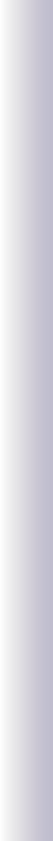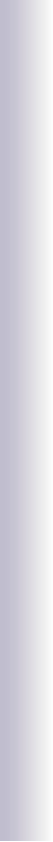# Code bomen

FraktalH

terug

//FraktalH  After Casey Reas and Ben Fry
// www.processing.org
void setup() {
size(400,400);
noLoop();
smooth();
}

void draw() {
background(255);
stroke(230);fill(230);
rect(20,80,160,240);
rect(220,80,160,240);
rect(170,180,60,40);
stroke(0);
// Go to the center of the screen
translate(width/2,height/2);
// Start the recursive branching!
branch(150);

}

void branch(float h) {
// Each branch will be 2/3rds the size of the previous one
h *= 0.66f;

// All recursive functions must have an exit condition!!!!
// Here, ours is when the length of the branch is 2 pixels or less
if (h > 1) {
pushMatrix();    // Save the current state of transformation (i.e. where are we now)
rotate(HALF_PI);   // Rotate by theta
line(0,0,0,-h);// Draw the branch
translate(0,-h); // Move to the end of the branch
branch(h);       // Ok, now call myself to draw two new branches!!
popMatrix();     // Whenever we get back here, we "pop" in order to restore the previous matrix state

// Repeat the same thing, only branch off to the "left" this time!
pushMatrix();
rotate(-HALF_PI);
line(0,0,0,-h);
translate(0,-h);
branch(h);
popMatrix();
}
}

Dichotomous tree

terug

// Dichotomous tree
// Code after Casey Reas and Ben Fry
// www.processing.org
float standoff=.6,factor=.75;
void setup() {
size(400,400);
}

void draw() {
noLoop();
background(255);
stroke(0,50);
float lengt=90;
translate(width/2,height-30);
// Draw a line 60 pixels
line(0,0,0,-lengt);
// Move to the end of that line
translate(0,-lengt);
// Start the recursive branching!
children(lengt);
}

void children(float lengt) {
// Each branch will be 2/3rds the size of the previous one
lengt *= factor;

// All recursive functions must have an exit condition!!!!
// Here, ours is when the length of the branch is 2 pixels or less
if (lengt > 2) {
pushMatrix();    // Save the current state of transformation (i.e. where are we now)
line(standoff*lengt,0,-standoff*lengt,0);
line(standoff*lengt,0,standoff*lengt,-lengt);
translate(standoff*lengt,-lengt); // Move to the end of the branch
children(lengt);       // Ok, now call myself to draw two new branches!!
popMatrix();     // Whenever we get back here, we "pop" in order to restore the previous matrix state

// Repeat the same thing, only branch off to the "left" this time!
pushMatrix();
line(-standoff*lengt,0,-standoff*lengt,-lengt);
translate(-standoff*lengt,-lengt);
children(lengt);
popMatrix();
}
}

Pythagoras

terug

//written by J.G.van Unnik, february 2011
void setup(){
size(400,350);
background(255);
stroke(0);
noFill();
noLoop();
}
void draw(){
float side=65;
translate((width-side)/2,height-40);
scale(1.0,-1.0);
rect(0,0,side,side);
translate(0,side);
pyth(side);
}
void pyth(float side){
if(side>2){
//draw two rectangles pythagoraswisely
pushMatrix();
//first rectangle
float newside=sin(QUARTER_PI)*side;
rotate(QUARTER_PI);
rect(0,0,newside,newside);
translate(0,newside);
pyth(newside);  //recursive call of sub pyth()
popMatrix();
//second rectangle
translate(side,0);
scale(-1,1);
rotate(QUARTER_PI);
rect(0,0,newside,newside);
translate(0,newside);
pyth(newside);
side=newside;
}
}

terug